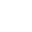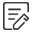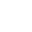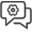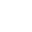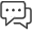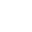RECOMMEND NEWSFEATURED PRODUCT

Date:2022-08-05 16:07:22

### What is Baud Rate? The Difference Between to Baud Rate, Bit Rate and Communication Speed

In the field of communication transmission, some proper terms are often heard, such as: baud rate, bit rate, etc. Most people may not understand its meaning. What is baud rate? This article will explain the baud rate and other related knowledge in detail.1. What is bit rate

Bit rate is also known as signaling rate and information transmission rate (referred to as information rate, information rate).

Its definition is: the amount of information transmitted per unit time (per second) of the communication line (or system), that is, the number of binary digits that can be transmitted per second, usually expressed in Rb, and its unit is bits per second (bit/s or b/ s, the English abbreviation is bps).

In a binary system, the information rate (bit rate) is equal to the signal rate (baud rate), for example, when the system transmits at 50 binary symbols per second, the information rate is 50bit/s, and the signal rate is also 50Bd (baud rate). ). In the case of no modulation, the bit rate is equal to the baud rate; with phase modulation technology, the bit rate is not equal to the baud rate. The sending device and the receiving device of the communication system must work under the same baud rate, otherwise there will be frame synchronization errors.

2. What is the baud rate

Baud rate is also known as code transmission rate, symbol transmission rate (referred to as symbol rate), signal transmission rate (referred to as signaling rate) or modulation rate.

Its definition is: the number of symbols (pulses) transmitted per unit time (per second) of the communication line (or system); or the number of transformations of the modulated signal waveform per unit time during the signal modulation process, usually expressed in RB, the unit is baud (Bd or Baud, the former specification). If 1 symbol is transmitted per second, it is called 1Bd; if the time length of 1 symbol is 200ms, then 5 symbols can be transmitted per second, then the symbol rate (baud rate) is 5Bd.

The baud rate (symbol rate) does not limit what kind of code unit it is, so the system of this code unit must be specified when the baud rate is given. For M-ary symbols, the relationship between bit rate (information rate) Rb and baud rate (symbol rate) RB is: Rb=RB·lbM

In the formula: lbM=log2M, which represents the logarithm of M with the base 2. Obviously, for binary symbols, since lb2=1, Rb=RB, that is, the baud rate and the bit rate are numerically equal, but the units are different, that is, the meanings of the two are different.

For example, if the baud rate is 600Bd, then in binary, the bit rate is also 600bit/s; in quaternary, since lb4=2, the bit rate is 1200bit/s. It can be seen that multiple bits can be transmitted in one symbol.

3. Data transfer rate

Data transfer rate is also known as data transfer rate and data transfer rate.

Its definition is: the number of characters transmitted per unit time (per second) of the communication line (or system); or the number of code groups (blocks) or the number of bits transmitted per unit time (per second). Its unit is character/second; or code group/second, bit/second (it can be seen that when the data transmission rate uses "bit/s" as the unit, it is equal to the bit rate).

For example, in a computer asynchronous serial communication system, the data transmission rate is 960 characters/s, and each character includes 1 start bit, 8 data bits, and 1 stop bit, then the corresponding bit rate is 10×960 Bit/s=9600bit/s=9600bit/s; because it is binary encoding, the corresponding baud rate is also 9600Bd.

4. The connection between baud rate and bit rate: baud, bits and symbols

Baud (Bd) is a unit of measurement used to measure the number of signal changes per second for devices such as modems, that is, the number of times the state of the communication line changes per second, not the amount of data transmitted. The word "Potter" comes from a French name - Baudot. He developed a coding scheme for the French telegraph system in 1877. If the modem transmits 1 bit (bit) of data every time the signal changes, then the bit rate (information rate) of the system is the same as the baud rate (symbol rate). However, after the coding technology is adopted, 1Bd (1 signal change) can be expressed as 2bit or more bits (bits). Each baud represents 2bit (bit) is called double-bit encoding, and each baud represents 3bit (bit) is called three-bit encoding. That is to say, one voltage (or current) waveform change may include several bits of data, therefore, baud rate and bit rate cannot be confused; the former refers to the number of voltage (or current) changes (changes), and the latter refers to The amount of data transferred.

A code cell is a digital unit that carries information, and refers to a waveform symbol that transmits a digital signal in a digital channel, that is, "a signal coding unit on the time axis". Symbols may be binary or multi-binary.

A bit is the measurement unit of "information amount", and the amount of information carried by one binary number is 1 bit (bit). For example, 10010110 is an 8-bit binary number, and the amount of information carried is 8 bits.

5. The difference between baud rate, bit rate and communication speed

There are many transmission-related proper terms in the field of communication, many of which are not particularly understood by laymen.

5.1 Concept

Bit rate: Refers to the number of bits (bits) transmitted per second. The unit is bps (Bit Per Second). The higher the bit rate, the more data is transmitted per second.

Baud rate: Indicates the number of symbols transmitted per second, which is an indicator to measure the data transmission rate.

In the information transmission channel, the signal unit that carries the data information is called the symbol, and the number of symbols transmitted through the channel per second is called the symbol transmission rate, or baud rate for short. The baud rate is an indicator of the bandwidth of the transmission channel.

Transmission rate: Transmission rate is a general term that refers to the rate at which data is transmitted from one point to another. Contains the above bit rate, baud rate, etc.

Communication speed: Communication speed and transmission speed are also a general term. For example, the I2C communication speed is 100KHz, and the SPI maximum communication speed supports 150Mbps.

5.2 Difference between bit rate and baud rate

5.2.1 Bit rate

Bit (bit) I believe everyone knows that 1 byte (Byte) is equal to 8 bits (bit). Naturally, bit rate is the number of bits transmitted per second.

5.2.2 Baud rate

In the field of electronic communication, Baud is the modulation rate, which refers to the rate at which the effective data signal modulates the carrier, that is, the number of times the modulation state of the carrier changes per unit time. It is a measure of the symbol transmission rate, 1 baud refers to the transmission of 1 symbol per second, and through different modulation methods, multiple bits of information can be loaded on one symbol symbol.

Similar to bit rate, you only need to understand the "baud" (ie symbol symbol) in baud rate as a transmission unit.

5.2.3 Relationship between baud rate and bit rate

Bit rate = baud rate x number of bits corresponding to a single modulation state.

1 Baud = log2M (bit/s)

where M is the number of coding levels of the signal.

Can also be written as: Rbit = Rbaud log2M

(Rbit: bit rate; Rbaud: baud rate)

It can be concluded that the bit rate of two-phase modulation (a single modulation state corresponds to 1 binary bit) is equal to the baud rate; the bit rate of four-phase modulation (a single modulation state corresponds to 2 binary bits) is twice the baud rate; eight The bit rate for phase modulation (3 binary bits for a single modulation state) is three times the baud rate; and so on.